# SBI PO Reasoning Questions 2019 (Day-40) High Level-New Pattern

Dear Aspirants, Our IBPS Guide team is providing new series of Reasoning Questions for SBI PO 2019 so the aspirants can practice it on a daily basis. These questions are framed by our skilled experts after understanding your needs thoroughly. Aspirants can practice these new series questions daily to familiarize with the exact exam pattern and make your preparation effective.

[WpProQuiz 6467]

Directions (1-4): Study the following information carefully and answer the below questions.

Seven person lives in seven floored building marked 1 to 7 in such a way that ground floor is marked 1 and topmost floor marked 7. Their first name is David, Manju, Sumit, Smith, Anuj, Vicky and Mike. Their surname is Khan, Rai, Singh, Ojha, Oberoi, Iyer and Sharma. Age of each person is different viz. 18, 23, 31, 32, 36, 41 and 54 years. All the information is not necessarily inthe same order.

Number of person below Khan’s floor is one less than number of person above Mike’s floor. Age of Sumit is 31 years and lives just below Iyer. Sharma neither lives on adjacent floor of Iyer nor lives on top floor. Age of Ojha is neither 18 years nor lives adjacent to Anuj. Iyer lives on even numbered floor at a gap of three floor from Anuj, whose last name is neither Singh nor Sharma. Oberoi lives just below Vicky, whose last name is neither Iyer nor lives above Anuj. Sharma lives two places above Smith, whose age is neither 31 years nor 54 years. Age of Singh is 23 years. Sum of ages of Khan and Anuj is equal to age of Oberoi. Difference between the age of Ojha and Oberoi is 23 years. David lives three places from the one whose age is 32 years. Two person lives between Singh and Oberoi. Rai lives on adjacent floor of the one whose age is 32 years. Age of Rai is neither 36 years nor 41 years.

1) Who among the following person lives just below the one whose age is 32 years?

a) Manju

b) Mike

c) Rai

d) Smith

e) None of these

2) Which of the following combination is true?

a) Rai – Vicky – 18

b) Singh – 7 – 32

c) Oberoi – Mike – 54

d) Anuj – Sharma – 23

e) David – 41 – Ojha

3) What is the last name of the one whose age is 41 years?

a) Iyer

b) Singh

c) Khan

d) Rai

e) None of these

4) How many person lives below the one whose last name is Rai?

a) Two

b) One

c) Three

d) Six

e) None of these

(Directions 5–7): A series of six two digit numbers isgiven as input. The further steps given are obtained by applying certain logic. Each step is a resultant of previous step only. Study the following information carefully and answer the questions given below it.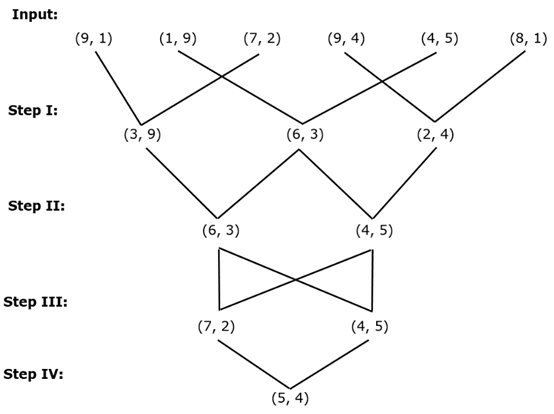As per above applied logic in above steps, find appropriate step for given input: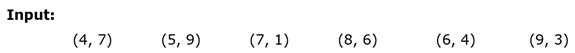5) What is the difference of lowest two digit number in step I and highest two digit number in step III?

a) 9

b) 7

c) 24

d) 16

e) None of these

6) Which of the following two digit number represents the final output?

a) 54

b) 30

c) 18

d) 24

e) None of these

7) What is the difference of sum of square of digits of two digit numbers in step II?

a) 32

b) 63

c) 45

d) 39

e) None of these

Directions (8-10): Study the following information carefully and answer the below questions.

Three friends Roy, Tom and Mike start walking towards town hall from their respective home. Point B is 28m south of point M. Tom standing at point A, walks 12m towards east to reach point E, takes a left turn of 24m to reach point L. Point G is 48m south of point J and is 28m east of point L. From point J, Tom takes a left turn of 16m to reach point H, which is 32m south of point C. Roy standing at point I, which is 28m east of point M. From point M, walks 28m towards his left to reach point B. Point D is 12m south of point F and is 16m east of point B. Mike standing at point P, walks 28m towards west to reach point N. He again takes a left turn of 36m to reach point Q, which is 18m east of town hall. Point K is 12m west of point C and is 48m north of town hall. Point F is 42m west of point Q.

8) What is the position of point L with respect to the town hall?

a) South-East

b) South

c) North- East

d) West

e) None of the above

9) What is the shortest distance between Roy’s initial position and town hall?

a) 18√2m

b) 14m

c) 20m

d) 16√3m

e) None of the above

10) Which of the following statement is not true?

a) Point D is south-west of point Q.

b) Point C is north-west of point J.

c) Point F is west of point G.

d) Roy is north of point F.

e) More than one statements are not true.

Direction (1-4) :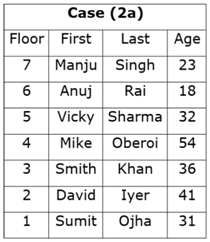We have:

• Iyer lives on even numbered floor at a gap of three floors from Anuj, whose last name is neither Singh nor Sharma, that means we have two possible places for Iyer, in case (1) Iyer lives on floor marked 6, in case (2) Iyer lives on floor marked 2.
• Age of Sumit is 31 years and lives just below Iyer.
• Sharma neither lives on adjacent floor of Iyer nor lives on top floor.
• Sharma lives two places above Smith, whose age is neither 31 years nor 54 years, that means in case (1) Sharma lives on floor marked 3, in case (2a) Sharma lives on floor marked 5, in case (2) Sharma lives on floor marked 4.

Based on above given information we have: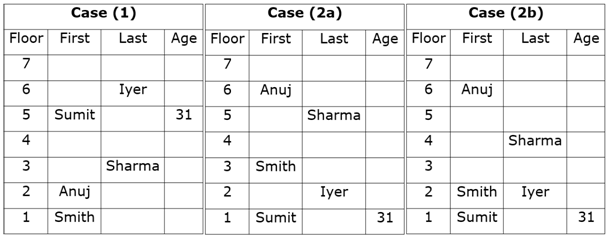Again, we have:

• Oberoi lives just below Vicky, whose last name is neither Iyer nor lives above Anuj, that means in case (2a) Vicky lives on floor marked 5, in case (2b)& case (2c) Vicky lives on floor marked 4 and case (1) is not valid.
• Difference between the age of Ojha and Oberoi is 23 years, thus only two such combinations are possible (18, 41) & (31, 54).
• Sum of ages of Khan and Anuj is equal to the age of Oberoi, thus only two such combinations are possible (18, 23, 41) & (18, 36, 54).
• Age of Ojha is neither 18 years nor lives adjacent to Anuj, since age of Oberoi is sum of two person thus, age of Oberoi must be either 41 years or 54 years.
• Since, age of Ojha is not 18 years, thus only possible age of Oberoi is 54 years and that of Ojha must be 31 years.

Based on above given information we have: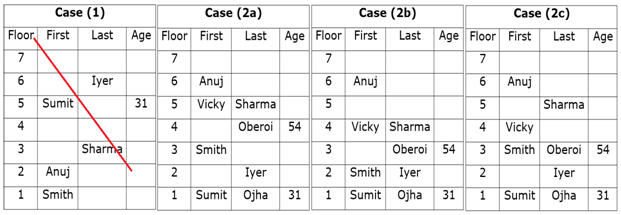Case (1) is not valid as Oberoi lives just below Vicky, whose last name is neither Iyer nor lives above Anuj.

Again, we have:

• Age of Singh is 23 years, as age of last name of Anuj is not Singh.
• Two person lives between Singh and Oberoi that means in case (2a) and case (2b) & case (2c) are not valid.
• Number of person below Khan’s floor is one less than number of person above Mike’s floor, which means Smith’s last name is Khan.
• Rai lives on adjacent floor of the one whose age is 32 years, that means Anuj’slast name is Rai and Sharma’s age is 32.
• David lives three places from the one whose age is 32 years, that means David lives at floor marked 2.
• Age of Rai is neither 36 years nor 41 years, which means age of Anuj must be 18 years.
• As, remaining person is Anuj and David, thus age of Anuj must be 36 years and age of David is 41 years.

Based on above given information we have final arrangement as follow: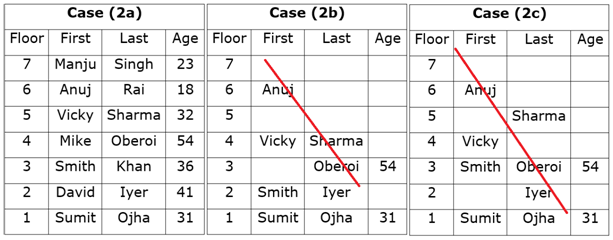Case (2b) &Case (2c) are not valid as two person lives between Singh and Oberoi.

Clearly, Mike lives just below the one whose age is 32 years.

Hence, option B is correct.

Clearly, combination C is correct.

Clearly, last name of the one whose age is 41 years is Iyer.

Hence, option A is correct.

Clearly, only five person lives below the one whose last name is Rai.

Hence, option E is correct.

Direction (5-7) :

We have: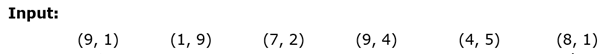Step I: In this step following logic is applied: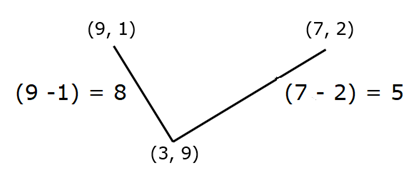Final result in step I, can be determined by difference of square of digits:

Result = (82 – 52) = 39

Step II: In this step following logic is applied: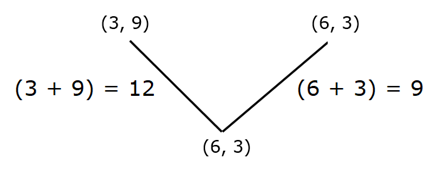Final result in step II, can be determined by difference of square of digits:

Result = (122 – 92) = 63

Step III: In this step following logic is applied: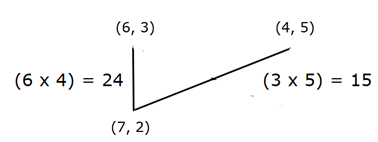Result in step III, can be determined by:

Result = (6 x 4)x 3 = 72

Similarly, (3x 15)x 3 = 45

Step IV: In this step following logic is applied: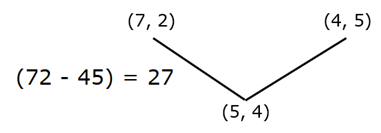Result in step IV, can be determined by:

Result = (27 x 2) = 54

Thus, after following above steps we have final output for the given input as: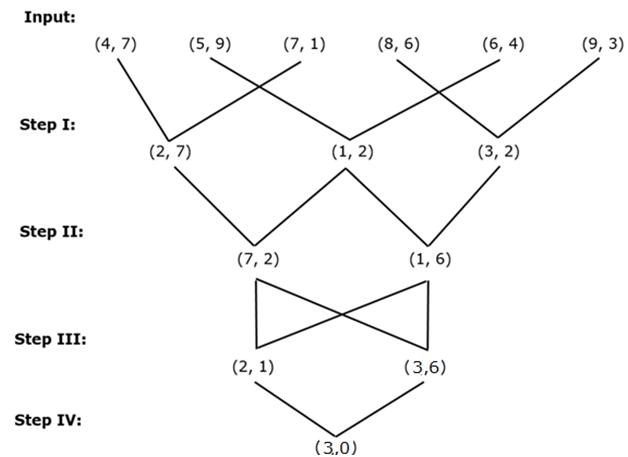Clearly, required difference = (36 – 12) = 24.

Hence, option C is correct.

Clearly, final output is 30.

Hence, option B is correct.

Clearly, required difference = (81 – 49) = 32.

Hence, option A is correct.

Direction (8-10) :

We have:

• Mike standing at point P, walks 28m towards west to reach point N.
• Point N is 36m north of point Q.
• Point Q is 18m east of town hall.
• Point F is 42m west of point Q, that means point F must be 24m west of town hall.

Based on above given information we have: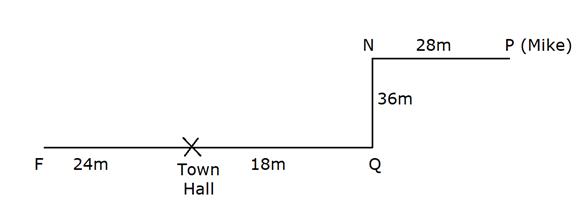Again, we have:

• Point D is 12m south of point F and is 16m east of point B, that means point F is 12m north of point D.
• From point M Roy walks 28m towards his left to reach point B.
• Roy standing at point I, which is 28m east of point M, that means point B is 28m north of point M.

Based on above given information we have: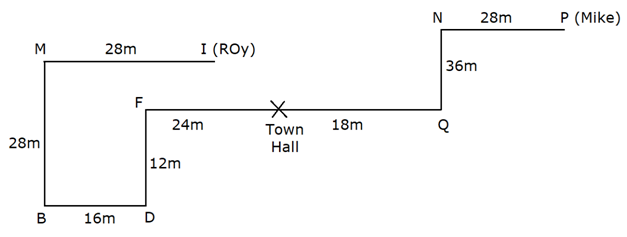Again, we have:

• Point K is 12m west of point C and is 48m north of town hall, that means point K is 48m north of town hall.
• Point H is 32m south of point C.
• From point J, Tom takes a left turn of 16m to reach point H.
• Point G is 48m south of point J and is 28m east of point L, that means point J is 16m east of point H.
• Tom standing at point A, walks 12m towards east to reach point E, takes a left turn of 24m to reach point L, which means point L is 24m north of point E.

Based on above given information we have final arrangement as follow: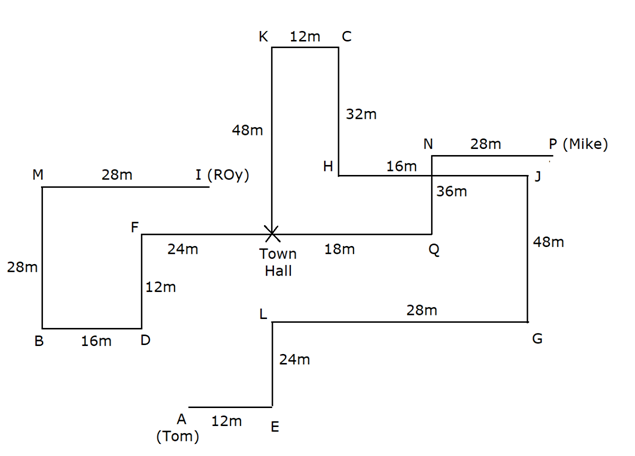Clearly, point L is south-east of town hall.

Hence, option A is correct.

We have: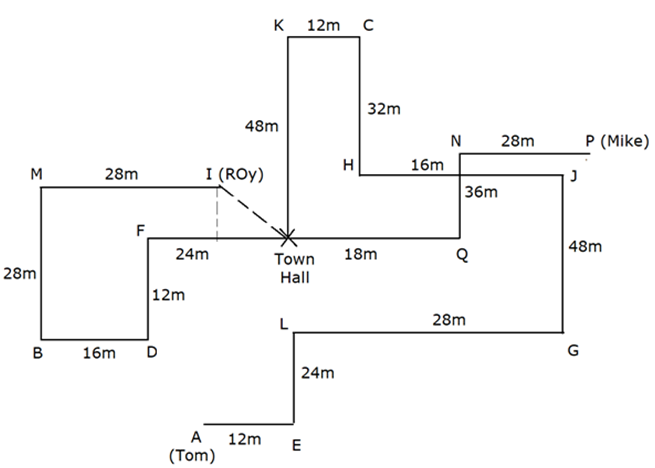Clearly, Base = (24 + 16) – 28 = 12m.

Perpendicular = (28 – 12) = 16m

Shortest distance = √(162 + 122) = 20m.

Hence, option C is correct.